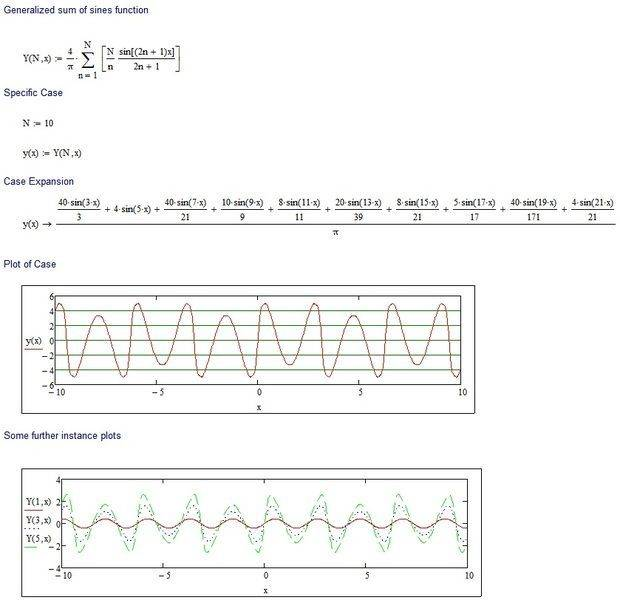# Programmable Graphing Calculator

back2square1
Hi, everyone.
I was wondering if you guys know a good *programmable* graphing calculator for Windows XP. I took a look at Microsoft Maths which was kinda fine but it lacked many feature (even though the input was neat). And then I came across GraphCalc (http://www.graphcalc.com/) which was really nice but I couldn't figure out many things. So please help to find a graphing calculator that can
- plot 2D as well as 3D functions
- plot piece-wise and periodic functions
- is programmable (or can be scripted)
I don't mean that I need source code and can program it to suite my need. I need that can plot functions like (Fourier Squarewave)

y=$\frac{4}{∏}$$\sum^{N}_{n}$$\frac{sin((2n+1)x)}{2n+1}$

by inputting something like

n=1;
f(x)=(4/∏)sin(x)/x;
while(n<N)
{
f(x)=f(x)+(4/∏)(sin((2n+1)x)/(2n+1))
n++
}
plot2d(f(x));
So that I can plot my squarewave to nth precision.

I've been manually converting them to this to plot my function

f(x)= (4sin(x)/pi) + (4sin(3x)/(3pi)) + (4sin(5x)/(5pi)) + (4sin(7x)/(7pi)) + (4sin(9x)/(9pi)) +(4sin(11x)/(11pi)) + (4sin(13x)/(13pi)) + (4sin(15x)/(15pi)) + (4sin(17x)/(17pi)) + (4sin(19x)/(19pi))

- has the ability to plot complex functions like f(z)=1/z
- polar and parametric equations

The programmable part is what I am looking the most because I need to plot a lot of Fourier Series waves.

If you guys know any such calculator, please help me. Thanks.

## Answers and Replies

DmytriE
I would recommend you use Matlab or Maple. Both are excellent "programmable" programs. I'm using the word "programmable" in the sense that you can store equations as variables names and write programmable files that can be run at a later time.

M Quack
Have you tried Gnuplot?

http://www.gnuplot.info/

I think it can do everything you require and then some. It also produces publication quality .pdf or .eps files.

Best of all, it is free.

What you want is a good maths software package with graphing capabilities. Don't restrict yourself by looking only at stuff that describes itself as a "graphing calculator".

Matlab and Maple mentioned above are highly recommended. But there's some really great freeware alternatives too.

My freeware picks would be gnu-Octave (a really excellent matlab clone) and Pylab (python with a bunch math and science packages bundled together). This is also a great way to learn the basics of python if you haven't already done so. (BTW, the "Enthought Python" freeware package is a convenient way to download and install this bundle).

Here is a screenshot.

#### Attachments

•pylab_scrsave.jpg
32.8 KB · Views: 401
back2square1
What you want is a good maths software package with graphing capabilities. Don't restrict yourself by looking only at stuff that describes itself as a "graphing calculator".

Matlab and Maple mentioned above are highly recommended. But there's some really great freeware alternatives too.

My freeware picks would be gnu-Octave (a really excellent matlab clone) and Pylab (python with a bunch math and science packages bundled together). This is also a great way to learn the basics of python if you haven't already done so. (BTW, the "Enthought Python" freeware package is a convenient way to download and install this bundle).

Here is a screenshot.

As you've done in the screenshot, Pylab seems to be exactly what I was looking for. So which one do you think would be better for me - gnu-Octave or Pylab? And do you know any GNU maths package cuz right now I'm running low on my funds.

Amd Maple is also *not* available for students!

As you've done in the screenshot, Pylab seems to be exactly what I was looking for. So which one do you think would be better for me - gnu-Octave or Pylab?
In the past I've preferred Octave because it's a more of a direct matlab replacement, and I am fairly familiar with matlab. Lately I've been giving pylab a trial, mostly to familiarize myself with python. Honestly they're both free so there's not real reason not to try them both and see which one you like.

And do you know any GNU maths package cuz right now I'm running low on my funds.
Amd Maple is also *not* available for students!

There are some gnu symbolic maths packages but I haven't used them for a while. Maybe someone else can recommend something here.

Python has a symbolic library (sympy) and an arbitrary precision one (mpmath) that makes it well rounded. See screen shot.

#### Attachments

NemoReally
For general plotting and easy entry of maths formula in a human readable form, try:

or
SMath - http://en.smath.info/forum/yaf_postst1447_SMath-Studio-0-95-4594--30-July-2012.aspx
or
Mathstudio - http://www.mathstudio.net/windows/

Mathcad is mainstream mathematical application in the Matlab/Maple/Mathematica class, and priced around $2000 (although there are educational and other discount / special offers / upgrades that significantly reduce the price). SMath is a free (donation) Mathcad "clone" - I put clone in quotes because although it has the same look and feel in many aspects, it is quite different in several significant ways. It is very capable but lacks the polish and coverage of Mathcad MathStudio (The Application formerly known as SpaceTime) is around$30 and quite clearly shows its PDA Calculator roots ... but don't let that put you off, it is a very sophisticated application indeed.

All three handle 2D and 3D functional plots, complex numbers, programming and, very usefully, symbolic maths.

I've attached an image of a quick Mathcad implementation of your equation. WYSIWYT ... there is no underlying programming you have to do to get the equations, you just pick the summation operator of a toolbar (or use the keyboard shortcut), type in the rest of expression and Robert is your parental sibling. The right arrow (→) invokes the symbolic processor, which then expands the sequence. Similarly, plots are created by toolbar or keyboard and then typing the function / variable name into an appropriated 'placeholder' in the plot. The text, equations and plots can be put just about anywhere on the page.